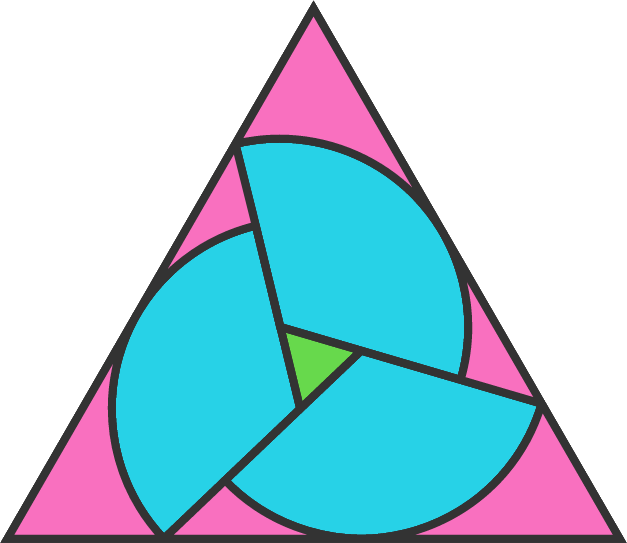# Slid sectors

Geometry Level 3A circle of radius $20$ is sliced into three congruent sectors, which are then slid apart to create a green equilateral triangle with side length $9.$ A larger equilateral triangle is then circumscribed.

The side length of this large triangle can be written as $\frac{a+b\sqrt{d}}{c}$ with $d$ square-free and $a, b, c$ irreducible.

What is the value of $a+b+c+d?$

×